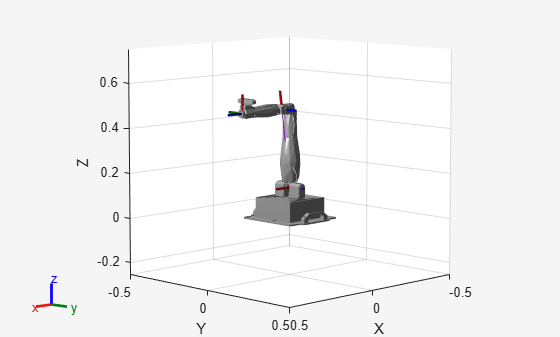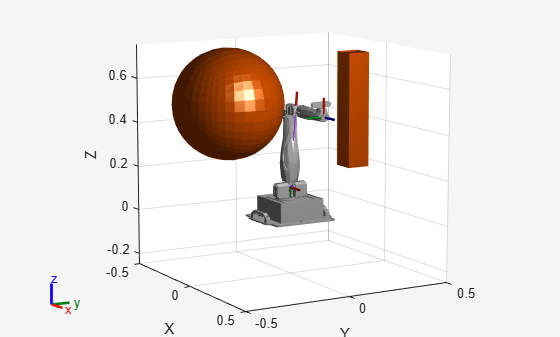# manipulatorStateSpace

State space for rigid body tree robot models

Since R2021b

## Description

The `manipulatorStateSpace` object represents the joint configuration state space of a rigid body tree robot model. For a given `rigidBodyTree` object, the nonfixed joints in the rigid body tree model form the state space. When sampling the state or specifying bounds, the values of the state vector correspond to joint positions that define a joint configuration with dimension equal to the `NumStateVariables` property.

Typically, the manipulator state space works with sampling-based path planners like the `plannerRRT` (Navigation Toolbox) and `plannerBiRRT` (Navigation Toolbox) objects. To sample and validate paths for manipulators, combine the state space with a state validator `manipulatorCollisionBodyValidator` object. Because the `manipulatorStateSpace` object derives from the `nav.StateSpace` (Navigation Toolbox) class, and is specified in the `StateSpace` property of the path planners.

To plan paths for manipulators using only Robotics System Toolbox™, see the `manipulatorRRT` object.

## Creation

### Syntax

``manipSS = manipulatorStateSpace``
``manipSS = manipulatorStateSpace(robot)``
``manipSS = manipulatorStateSpace(robot,numStateVariables)``

### Description

````manipSS = manipulatorStateSpace` creates a default state space for a rigid body tree with two revolute joints.```

example

````manipSS = manipulatorStateSpace(robot)` creates a state space for the specified `rigidBodyTree` object, `robot`.```
````manipSS = manipulatorStateSpace(robot,numStateVariables)` specifies the number of state variables, which is the number of nonfixed joints in the robot model. You must use this syntax for code generation. ```

## Properties

expand all

Rigid body tree robot model, specified as a `rigidBodyTree` object. After you create the `manipulatorStateSpace` object, this property is read-only.

Name of the state space object, specified as a string scalar or character vector.

Example: `"customManipulatorState"`

Dimension of the state space, specified as a positive numeric integer. This property is the dimension of the state space and should match the size of the robot model joint configuration. To get a joint configuration, see the `homeConfiguration` or `randomConfiguration` function.

After you create the object, this property is read-only.

Min and max bounds of the joint positions, specified as an n-by-2 matrix with rows of form `[min max]`. n is the number of state variables in the joint configuration space, specified in the `NumStateVariables` property. You must specify the ```[min max]``` joint positions in meters for prismatic joints and in radians for revolute joints.

Example: `[-10 10; -10 10; -pi pi]`

Data Types: `double`

## Object Functions

 `distance` Distance between states `enforceStateBounds` Limit state to state space bounds `sampleUniform` Sample state using uniform distribution `sampleGaussian` Sample state using Gaussian distribution `interpolate` Interpolate between states

## Examples

collapse all

Generate states to form a path, validate motion between states, and check for self-collisions and environmental collisions with objects in your world. The `manipulatorStateSpace` object represents the joint configuration space of your rigid body tree robot model, and can sample states, calculate distances, and enforce state bounds. The `manipulatorCollisionBodyValidator` object validates the state and motion based on the collision bodies in your robot model and any obstacles in your environment.

Use the `loadrobot` function to access predefined robot models. This example uses the Quanser QArm™ robot and joint configurations are specified as row vectors.

```robot = loadrobot("quanserQArm",DataFormat="row"); figure(Visible="on") show(robot); xlim([-0.5 0.5]) ylim([-0.5 0.5]) zlim([-0.25 0.75]) hold on```Configure State Space and State Validation

Create the state space and state validator from the robot model.

```ss = manipulatorStateSpace(robot); sv = manipulatorCollisionBodyValidator(ss,SkippedSelfCollisions="parent");```

Set the validation distance to `0.05`, which is based on the distance normal between two states. You can configure the validator to ignore self collisions to improve the speed of validation, but must consider whether your robot model has the proper joint limit settings set to ensure it does not collide with itself.

```sv.ValidationDistance = 0.05; sv.IgnoreSelfCollision = true;```

Place collision objects in the robot environment. Set the `Environment` property of the collision validator object using a cell array of objects.

```box = collisionBox(0.1,0.1,0.5); % XYZ Lengths box.Pose = trvec2tform([0.2 0.2 0.5]); % XYZ Position sphere = collisionSphere(0.25); % Radius sphere.Pose = trvec2tform([-0.2 -0.2 0.5]); % XYZ Position env = {box sphere}; sv.Environment = env;```

Visualize the environment.

```for i = 1:length(env) show(env{i}) end view(60,10)```Plan Path

Start with the home configuration as the first point on the path.

```rng(0); % Repeatable results start = homeConfiguration(robot); path = start; idx = 1;```

Plan a path using these steps, in a loop:

• Sample a nearby goal configuration, using the Gaussian distribution, by specifying the standard deviation for each joint angle.

• Check if the sampled goal state is valid.

• If the sampled goal state is valid, check if the motion between states is valid and, if so, add it to the path.

```for i = 2:25 goal = sampleGaussian(ss,start,0.25*ones(4,1)); validState = isStateValid(sv,goal); if validState % If state is valid, check motion between states. [validMotion,~] = isMotionValid(sv,path(idx,:),goal); if validMotion % If motion is valid, add to path. path = [path; goal]; idx = idx + 1; end end end```

Visualize Path

After generating the path of valid motions, visualize the robot motion. Because you sampled random states near the home configuration, you should see the arm move around that initial configuration.

To visualize the path of the end effector in 3-D, get the transformation, relative to the base world frame at each point. Store the points as an xyz translation vector. Plot the path of the end effector.

```eePose = nan(3,size(path,1)); for i = 1:size(path,1) show(robot,path(i,:),PreservePlot=false); eePos(i,:) = tform2trvec(getTransform(robot,path(i,:),"END-EFFECTOR")); % XYZ translation vector plot3(eePos(:,1),eePos(:,2),eePos(:,3),"-b",LineWidth=2) drawnow end```## Version History

Introduced in R2021b